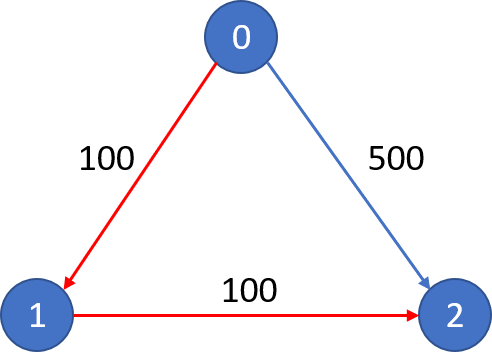## 算法分析课程博客分享 7

### 513. 找树左下角的值(Find Bottom Left Tree Value)

2
/ \
1   3

1

1
/ \
2   3
/   / \
4   5   6
/
7

7

``````int findBottomLeftValue(TreeNode* root) {
queue<TreeNode*> q{ {root} };
int ans = 0;
while (!q.empty()) {
ans = q.front()->val;

int size = q.size();
for (int i = 0; i < size; ++i) {
TreeNode* cur = q.front();
q.pop();
if (cur->left != NULL) {
q.push(cur->left);
}
if (cur->right != NULL) {
q.push(cur->right);
}
}
}

return ans;
}
``````

### 787. K 站中转内最便宜的航班(Cheapest Flights Within K Stops)

n = 3, edges = [[0,1,100],[1,2,100],[0,2,500]]
src = 0, dst = 2, k = 1从城市 0 到城市 2 在 1 站中转以内的最便宜价格是 200，如图中红色所示。

n = 3, edges = [[0,1,100],[1,2,100],[0,2,500]]
src = 0, dst = 2, k = 0从城市 0 到城市 2 在 0 站中转以内的最便宜价格是 500，如图中蓝色所示。

• n 范围是 [1, 100]，城市标签从 0 到 n - 1.
• 航班数量范围是 [0, n * (n - 1) / 2].
• 每个航班的格式 (src, dst, price).
• 每个航班的价格范围是 [1, 10000].
• k 范围是 [0, n - 1].
• 航班没有重复，且不存在环路

``````int findCheapestPrice(int n, vector<vector<int>>& flights, int src, int dst, int K) {
vector<vector<pair<int, int>>> edges(n, vector<pair<int, int>>());
for (vector<int> e : flights) {
edges[e].push_back(make_pair(e, e));
}

queue<pair<int, int>> q{ {make_pair(src, 0)} };
int cost = INT_MAX;
for (int i = 0; !q.empty() && i <= K; ++i) {
int size = q.size();
for (int j = 0; j < size; ++j) {
pair<int, int> u = q.front();
q.pop();
for (pair<int, int> e : edges[u.first]) {
if (e.first == dst) {
cost = min(cost, u.second+e.second);
} else if (cost > u.second+e.second) {
q.push(make_pair(e.first, u.second+e.second));
}
}
}
}

return (cost == INT_MAX) ? -1 : cost;
}
``````

``````int findCheapestPrice(int n, vector<vector<int>>& flights, int src, int dst, int K) {
vector<int> dist(n, 1e9);
dist[src] = 0;
for (int i = 0; i <= K; ++i) {
vector<int> tmp = dist;
for (vector<int> f : flights) {
tmp[f] = min(tmp[f], dist[f]+f);
}
dist = tmp;
}

return (dist[dst] >= 1e9) ? -1 : dist[dst];
}
``````

### 815. 公交路线(Bus Routes)

s

routes = [[1, 2, 7], [3, 6, 7]]
S = 1
T = 6

• 1 <= routes.length <= 500.
• 1 <= routes[i].length <= 500.
• 0 <= routes[i][j] < 10 ^ 6.

``````int numBusesToDestination(vector<vector<int>>& routes, int S, int T) {
if (S == T)
return 0;

unordered_map<int, unordered_set<int>> path;
for (int i = 0; i < routes.size(); ++i) {
for (int src : routes[i]) {
path[src].insert(i);
}
}

queue<int> q{ {S} };
int dist = 1;
while (!q.empty()) {
int size = q.size();
for (int i = 0; i < size; ++i) {
int src = q.front();
q.pop();
for (int bus : path[src]) {
for (int dst : routes[bus]) {
if (dst == T)
return dist;
q.push(dst);
}
routes[bus].clear();
}
path[src].clear();
}
++dist;
}

return -1;
}
``````